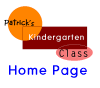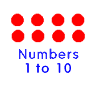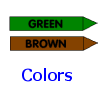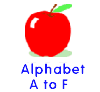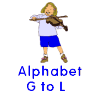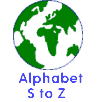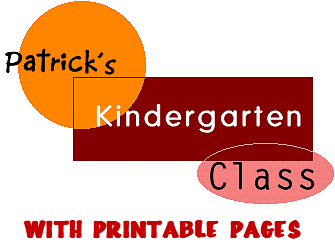Numbers

 1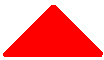Here is 1 triangle 2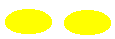Here are 2 ovals 3Here are 3 rectangles 4Here are 4 circles 5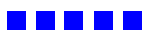Here are 5 squares 6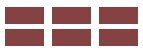Here are 6 rectangles 7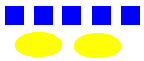5 squares and 2 ovals make 7 8Here are 8 circles 95 squares and 4 circles make 9 10Here are 10 squares